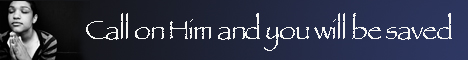Note:  Do not rely on this information. It is very old.

# Parallelogramof Velocities

Parallelogram of Velocities. If a body at B have a velocity represented by b a, it will travel over the distance B a in one second. Similarly, if it have a velocity represented by B D, it will travel to d in one second. If, however, the body tend to move with both these velocities at the same time, it will reach the point c at the end of a second, a c and n c being drawn parallel to B d and B A. Its path can also be shown to have been the diagonal B c; in fact, the body moves exactly as though it had the velocity represented by B C. This can be experimentally illustrated by letting a marble move along a groove in the direction B A, while at the same time the board containing the groove is moved in the direction B D. The groove will then lie along D C, so that the marble will be at C. The proposition of the parallelogram can hence be stated in these terms: - If a body tend to move with two uniform velocities, which can be represented in magnitude and direction by the two sides of a parallelogram drawn through any point B, the resultant velocity will be represented by that diagonal of the parallelogram which passes through B.